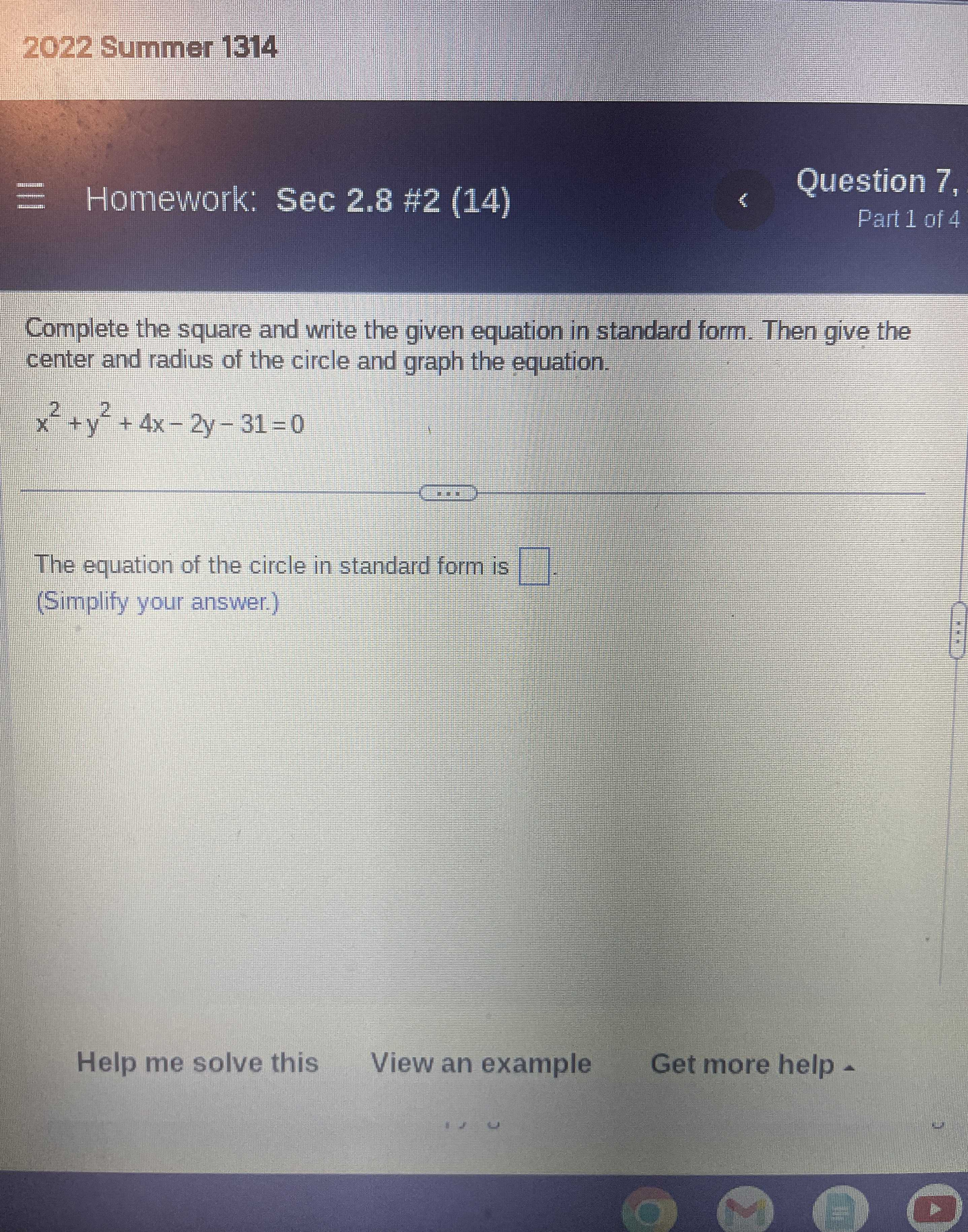### ¿Todavía tienes preguntas de matemáticas?

Pregunte a nuestros tutores expertos
Algebra
PreguntaComplete the square and write the given equation in standard form. Then give the center and radius of the circle and graph the equation.

$$x ^ { 2 } + y ^ { 2 } + 4 x - 2 y - 31 = 0$$

The equation of the circle in standard form is (Simplify your answer.)

$$(x+ 2)^{2}+ (y- 1)^{2} = 36$$
Center is $$(- 2,1)$$